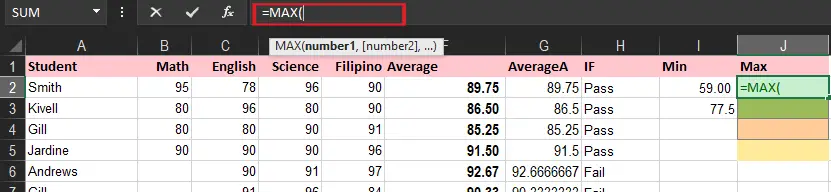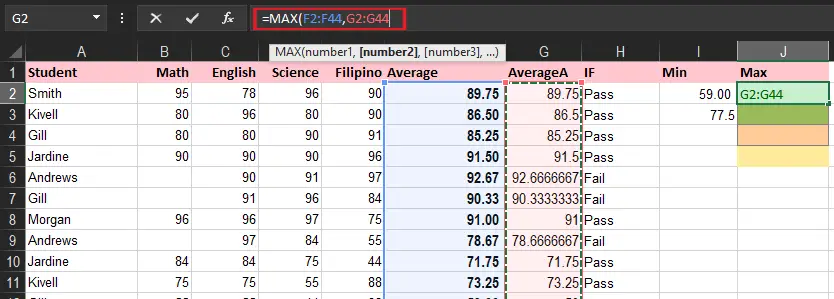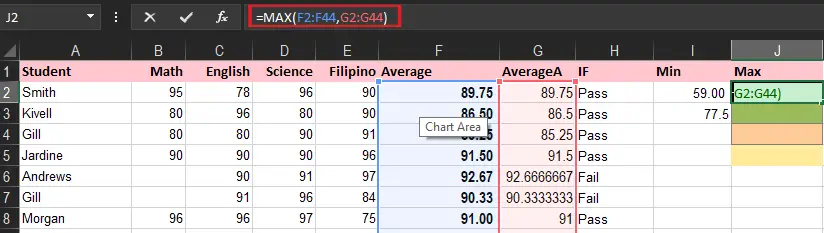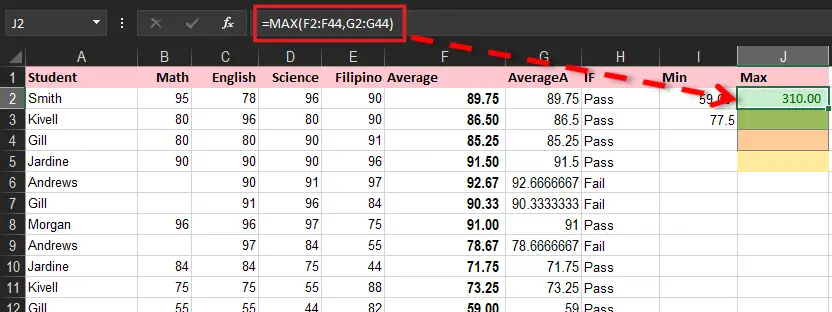# How To Use Max Function In Excel With Examples and Tricks

This tutorial will explain the Max function of Excel how to use it along with the examples and tricks which will make the discussion easier to understand. Furthermore, this function will highlight and give the highest number of the specified value.

As an overview, this function is not only easy to use but also straightforward functions. Despite that, it is easy to use there is still a scheme that is advantageous in working out worksheets.

Let’s get started and see how it is capable of having conditions, thus how it’s accurate in evaluating the largest number or value.

## What is MAX Function in Excel?

The Max Function in Excel is a function that returns the largest value from the data provided. Finding of the maximum set of data/arrays is easy with help of this function. Besides that, it is under the built-in function category of statistical functions.

Therefore, max excel functions will only count number values but ignore string characters, empty cells, and logical functions such as TRUE or False.

For example, you want to get the maximum scores for the student in all subjects. This is perfectly suited to execute this task.

### MAX formula in Excel

The syntax of this formula looks like below:

`=max (number1, [number2,..number_n])`

### Return Value

The maximum value contains in the given data.

### Arguments

`number1`: A required argument that could be a number, range, or reference cell containing a numerical value.

`number2`: Optional. Similarly, number, reference of cell or range that includes numerical data.

## Max function in Microsoft Excel Versions

Microsoft Excel Version 365 excel, excel 2010 excel 2007, excel 2013, excel 2016 and excel 2019 excel Max function is still available. Thus, even on the lower versions, it is still functional.

## What does the MAX function do in Excel?

Generally, the max formula returns the largest numeric value supplied. However to visualize this task let’s take this example of how it does.

These are the important things to be keep in mind in utilizing this function:

• It caters to arguments like numbers, references, arrays, or cells containing numbers.
• All directly input text which represents numbers and logical values are still counted.
• Only numbers that are arrays or references are only used in arguments. Hence, logical values, empty cells, and text are ignored in the array.
• Max returns zero if arguments do not contain numbers.
• It returns Error value when the arguments only contain text which cannot represent numbers.
• Use the MAXA function if logical values and text representations of numbers.

## How to Use MAX function to returns the largest number

This time we’ll assume the student’s grades to locate the maximum score using the max excel function. Examine the following given data as we apply this formula.

Let’s apply this formula:` `=MAX(F2:F44)

It returns the maximum value of the students average. Now let’s take a look at the next scenario and find how it differs or similar to the illustration above.

### Find highest value in non-adjacent cells or ranges

Time needed: 3 minutes

The MAX function also covers finding the highest value in non-adjacent cells or ranges. The only thing you should do is to contain references to individual cells or ranges.

Here are the following steps to do it seamlessly:

1. First Enter the MAX formula in the specified cell =MAX(2. Then, after the open parenthesis, select the range of cells you want to evaluate and separate it with a comma.3. After that, type the closing parenthesis.4. Lastly, Hit Enter key to complete the formula.## Summary

In summary, we analyzed the Max function in Excel which we learned its beneficial especially when we are finding the largest values in our data. Considering it is an explicit function it’s advantageous when we are analyzing data, particularly the grading sheet of students.

1.